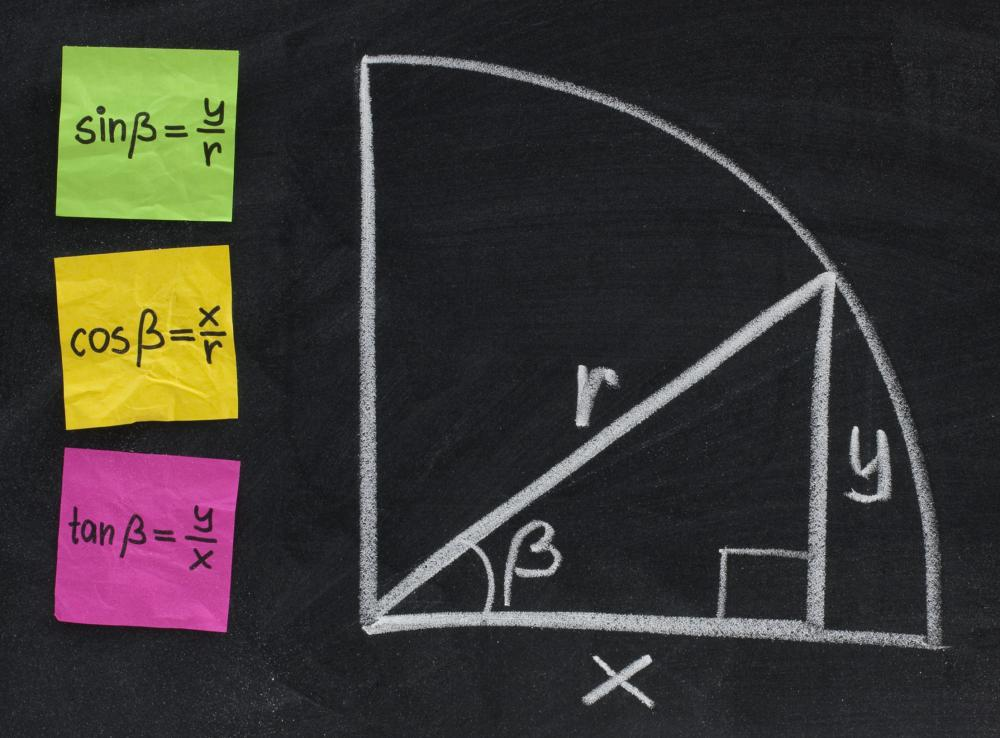# What is the Cosine Rule?

G. Wiesen
G. WiesenThe cosine rule is used in trigonometry.

The cosine rule is a formula commonly used in trigonometry to determine certain aspects of a non-right triangle when other key parts of that triangle are known or can otherwise be determined. It is an effective extension of the Pythagorean theorem, which typically only works with right triangles and states that the square of the hypotenuse of the triangle is equal to the squares of the other two sides when added together (c2=a2+b2). The cosine rule is an extension of this mathematic principal that makes it effective for non-right triangles and states that in regard to a certain angle, the square of the side of the triangle opposite that angle is equal to the squares of the other two sides added together, minus two times both of those sides multiplied together with the cosine of that angle (c2=a2+b2-2ab cosC where C is the angle opposite side c).The Cosine Rule is an effective extension of the Pythagorean theorem.

Though many modern mathematical sources give credit to a Muslim mathematician named al-Kashi for the creation of the cosine rule, there is also some evidence to indicate that the ancient Greek mathematician Euclid had devised a similar principal. Much of modern algebra and trigonometry comes from the efforts of Muslims during the European Dark Ages, and it was around the 15th century that al-Kashi codified the formula in a way that is still understood today. In France the rule is even referred to as Le théorème d’Al-Kashi or “the theorem of al-Kashi.”

In general, the cosine rule is used in triangulation and a number of other practical applications of trigonometry. It is especially useful in systems where the lengths all three sides are known or can be established and the measure of the angles within the triangle need to be determined. The cosine rule can also be used to establish the length of one side of a triangle if the lengths of the other two sides are known as well as the angle opposite that side.

Since the cosine rule deals with triangles consisting of three straight sides and their angles, it generally only works within the realm of Euclidean geometry. Different versions of the cosine rule can be used for non-Euclidean geometry such as spherical geometry and hyperbolic geometry. In these systems, a triangle is established by three points in curved space and the lines, usually curved lines, which connect them. The hyperbolic law of cosines and the spherical law of cosines function much like the Euclidean cosine rule, in that they can allow someone to establish the three angles of a triangle as long as he or she knows the three sides. Unlike Euclidean cosine rules, however, these non-Euclidean laws can also allow someone to determine the sizes of the three sides of a triangle if he or she knows the three angles.

## You might also Like## Discuss this Article

Forgot password?
###### Register:
•The cosine rule is used in trigonometry.
•The Cosine Rule is an effective extension of the Pythagorean theorem.